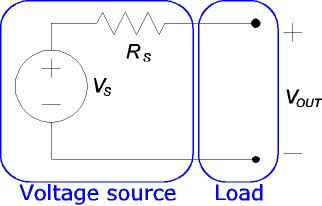Updated onAn electrical load is an electrical component or portion of a circuit that consumes electric power. This is opposed to a power source, such as a battery or generator, which produces power. In electric power circuits examples of loads are appliances and lights. The term may also refer to the power consumed by a circuit.

The term is used more broadly in electronics for a device connected to a signal source, whether or not it consumes power. If an electric circuit has an output port, a pair of terminals that produces an electrical signal, the circuit connected to this terminal (or its input impedance) is the load. For example, if a CD player is connected to an amplifier, the CD player is the source and the amplifier is the load.

Load affects the performance of circuits with respect to output voltages or currents, such as in sensors, voltage sources, and amplifiers. Mains power outlets provide an easy example: they supply power at constant voltage, with electrical appliances connected to the power circuit collectively making up the load. When a high-power appliance switches on, it dramatically reduces the load impedance.

If the load impedance is not very much higher than the power supply impedance, the voltages will drop. In a domestic environment, switching on a heating appliance may cause incandescent lights to dim noticeably.

## A more technical approach

When discussing the effect of load on a circuit, it is helpful to disregard the circuit's actual design and consider only the Thévenin equivalent. (The Norton equivalent could be used instead, with the same results.) The Thévenin equivalent of a circuit looks like this:

With no load (open-circuited terminals), all of V S falls across the output; the output voltage is V S . However, the circuit will behave differently if a load is added. We would like to ignore the details of the load circuit, as we did for the power supply, and represent it as simply as possible. If we use an input resistance to represent the load, the complete circuit looks like this:

Whereas the voltage source by itself was an open circuit, adding the load makes a closed circuit and allows charge to flow. This current places a voltage drop across R S , so the voltage at the output terminal is no longer V S . The output voltage can be determined by the voltage division rule:

V O U T = V S R L R L + R S

If the source resistance is not negligibly small compared to the load impedance, the output voltage will fall.

This illustration uses simple resistances, but similar discussion can be applied in alternating current circuits using resistive, capacitive and inductive elements.

## References

Similar Topics
Anne Lise Bakken
Eric Martin (linebacker)
Sidhant Gupta
Topics